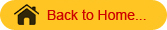## STAM101 :: Lecture 18 :: Factorial experiments- factor and levels – types – symmetrical and asymmetrical – simple, main and interaction effects – advantages and disadvantages

Factorial Experiments: When two or more number of factors are investigated simultaneously in a single experiment such experiments are called as factorial experiments.

Terminologies
1. Factor: Factor refers to a set of related treatments. We may apply of different doses of nitrogen to a crop. Hence nitrogen irrespective of doses is a factor.
1. Levels of a factor: Different states or components making up a factor are known as the levels of that factor. eg different doses of nitrogen.

Types of factorial Experiment

A factorial experiment is named based on the number of factors and levels of factors. For example, when there are 3 factors each at 2 levels the experiment is known as 2 X 2 X 2 or 23 factorial experiments.

If there are 2 factors each at 3 levels then it is known as 3 X 3 or 32 factorial experiment.

• In general if there are n factors each with p levels then it is known as pn factorial experiment.

• For varying number of levels the arrangement is described by the product. For example, an experiment with 3 factors each at 2 levels, 3 levels and 4 levels respectively then it is known as 2 X 3 X 4 factorial experiment.
• If all the factors have the same number of levels the experiment is known as symmetrical factorial otherwise it is called as mixed factorial.

• Factors are represented by capital letters. Treatment combinations are usually by small letters.
• For example, if there are 2 varieties v0 and v1 and 2 dates of sowing d0 and d1 the treatment combinations will be

• vodo, v1do, v1do and v1d1.

Simple and Main Effects

Simple effect of a factor is the difference between its responses for a fixed level of other factors.

Main effect is defined as the average of the simple effects.

Interaction is defined as the dependence of factors in their responses.  Interaction is measured as the mean of the differences between simple effects.

1. In such type of experiments we study the individual effects of each factor and their interactions.
2. In factorial experiments a wide range of factor combinations are used.
3. Factorial approach will result in considerable saving of the experimental resources, experimental material and time.